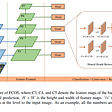# Intuition

Let’s assume we only have a feature map detecting the right eye of a face. Can we use it to locate a face? It should. Since the right eye should be on the top-left corner of a facial picture, we can use that to locate the face easily.

Nevertheless, a feature map rarely gives you such precise answer. But if we have other feature maps specialized in detecting the left eye, the nose or the mouth, we can combine information together to make face detection easier and more accurate. To generalize this solution, we create 9 region-based feature maps each detecting the top-left, top-middle, top-right, middle-left, … or bottom-right area of an object. By combing the votes from these feature maps, we determine the class and the location of the objects.

# Motivations

R-CNN based detectors, like Fast R-CNN or Faster R-CNN, process object detection in 2 stages.

• Generate region proposals (ROIs), and
• Make classification and localization (boundary boxes) predictions from ROIs.

The Fast R-CNN and Faster R-CNN program flow are summarized as:

`feature_maps = process(image)ROIs = region_proposal(feature_maps)for ROI in ROIs    patch = roi_pooling(feature_maps, ROI)    class_scores, box = detector(patch)    class_probabilities = softmax(class_scores)`

Fast R-CNN computes the feature maps from the whole image once. It then derives the region proposals (ROIs) from the feature maps directly. For every ROI, no more feature extraction is needed. That cuts down the process significantly as there are about 2000 ROIs. Following the same logic, R-FCN improves speed by reducing the amount of work needed for each ROI. The region-based feature maps are independent of ROIs and can be computed outside each ROI. The remaining work, which we will discuss later, is much simpler and therefore R-FCN is faster than Fast R-CNN or Faster R-CNN. Here is the pseudo code for R-FCN for comparison.

`feature_maps = process(image)ROIs = region_proposal(feature_maps)         score_maps = compute_score_map(feature_maps)for ROI in ROIs    V = region_roi_pool(score_maps, ROI)         class_scores, box = average(V)                   # Much simpler!    class_probabilities = softmax(class_scores) `

# R-FCN

Let’s get into the details and consider a 5 × 5 feature map M with a square object inside. We divide the square object equally into 3 × 3 regions. Now, we create a new feature map from M to detect the top left (TL) corner of the square only. The new feature map looks like the one on the right below. Only the yellow grid cell [2, 2] is activated.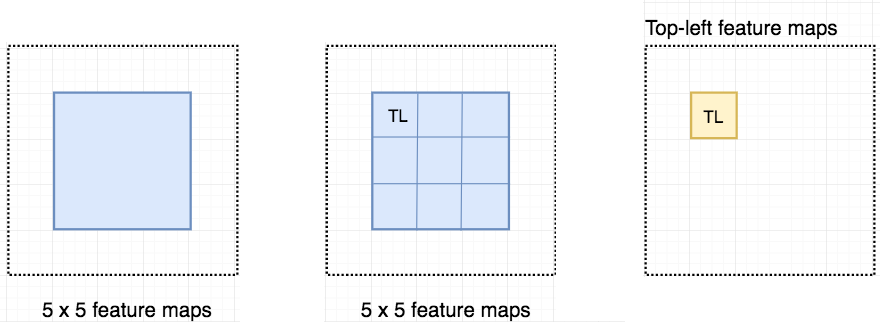Create a new feature map from the left to detect the top left corner of an object.

Since we divide the square into 9 parts (top-left TR, top-middle TM, top-right TR, center-left CF, …, bottom-right BR), we create 9 feature maps each detecting the corresponding region of the object. These feature maps are called position-sensitive score maps because each map detects (scores) a sub-region of the object.

Let’s say the dotted red rectangle below is the ROI proposed. We divide it into 3 × 3 regions and ask how likely each region contains the corresponding part of the object. For example, how likely the top-left ROI region contains the left eye. We store the results into a 3 × 3 vote array in the right diagram below.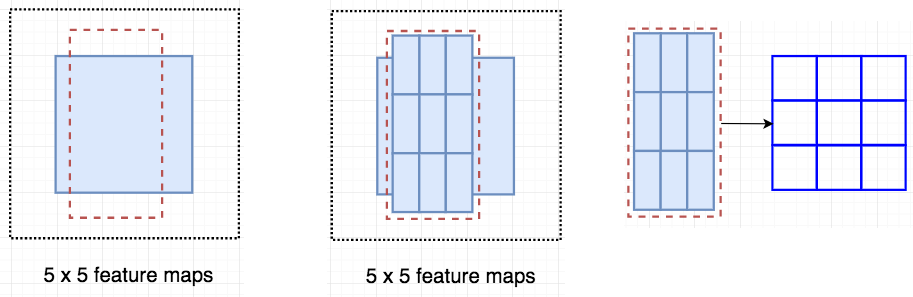Apply ROI onto the feature maps to output a 3 x 3 array.

This process to map score maps and ROIs to the vote array is called position-sensitive ROI-pool which is very similar to the ROI pool in the Fast R-CNN.

For the diagram below:

• We take the top-left ROI region, and
• Map it to the top-left score map (top middle diagram).
• We compute the average score of the top-left score map bounded by the top-left ROI (blue rectangle). About 40% of the area inside the blue rectangle has 0 activation and 60% have 100% activation, i.e. 0.6 in average. So the likelihood that we have detected the top-left object is 0.6.
• We store the result (0.6) into array
• We redo it with the top-middle ROI but with the top-middle score map now.
• The result is computed as 0.55 and stored in array. This value indicates the likelihood that we detected the top-middle object.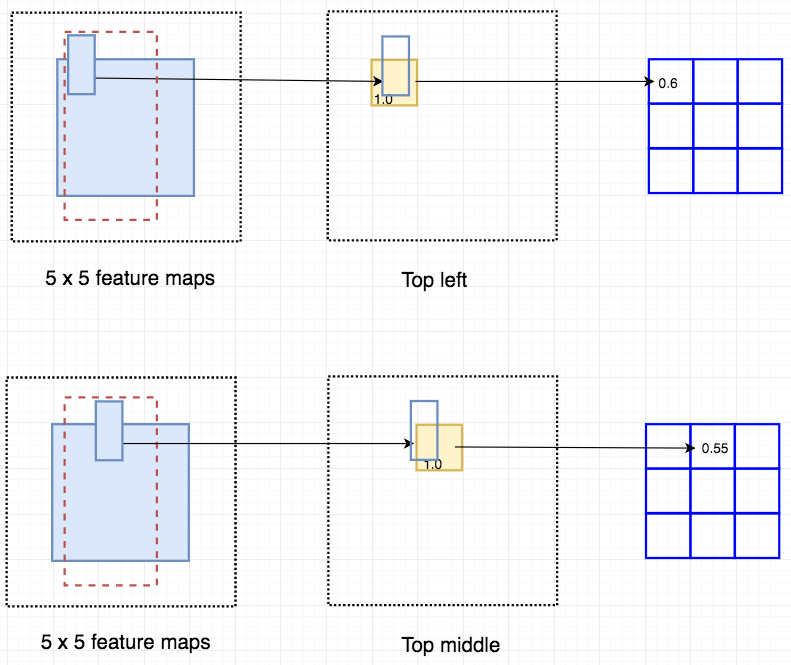Overlay a portion of the ROI onto the corresponding score map to calculate V[i][j]

After calculating all the values for the position-sensitive ROI pool, the class score is the average of all its elements.

Let’s say we have C classes to detect. We expand it to C + 1 classes so we include a new class for the background (non-object). Each class will have its own 3 × 3 score maps and therefore a total of (C+1) × 3 × 3 score maps. Using its own set of score maps, we predict a class score for each class. Then we apply a softmax on those scores to compute the probability for each class.

Let’s see a real example. Below, we have 9 score maps in detecting the top-left to the bottom-right region of a baby. In the top diagram, the ROI aligns well with the ground truth. The solid yellow rectangle in the middle column indicates the ROI sub-region corresponding to the specific score map. Activations are high inside the solid yellow box for every score maps. Therefore the scores in the vote array are high and a baby is detected. In the second diagram, the ROI is misaligned. The score maps are the same but the corresponding locations for the ROI sub-regions (solid yellow) are shifted. The overall activations are low and we will not classify this ROI contains a baby.

Below is the network flow for R-FCN. Instead of dividing ROIs into 3 × 3 regions and a 3× 3 ROI pool, we generalize them into k× k. i.e. we will need k× k × (C+1) score maps. Therefore, R-FCN takes in feature maps and apply convolution to create position-sensitive score maps with depth k× k × (C+1). For each ROI, we apply the position-sensitive ROI pool to generate the k× k vote array. We average the array and use softmax to classify the object.

Here is the data flow for the R-FCN.

# Boundary box regression

We apply convolution filters to create the k× k × (C+1) score maps for classification. To perform the boundary box regression, the mechanism is almost the same. We use another convolution filters to create a 4×k× k map from the same feature maps. We apply the position-based ROI-pool to compute a k×k array with each element containing a boundary box. The final prediction is the average of those elements.

# Results

Both Faster R-CNN +++ and R-FCN use ResNet-101 for feature extraction. (R-FCN uses multi-scale training.)

R-FCN demonstrates 20x faster than the Faster R-CNN.

# Credit & reference

R-FCN: Object Detection via Region-based Fully Convolutional Networks

--

--

--

## More from Jonathan Hui

Deep Learning

Love podcasts or audiobooks? Learn on the go with our new app.

## A brief introduction to spaCy using python: Production grade NLP library## How to prepare for a “Data Science” Interview?## Winner Interview with Shivam Bansal | Data Science for Good Challenge: City of Los Angeles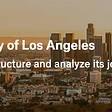## Accessing the Twitter API with Tweepy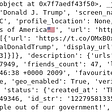## 5 Things You Need to Know Before Applying to Data Science Master’s Programs## How to use OECD data in Python## So you are now a Data Science Manager… Now what?## In Search of Property listings in Nairobi.Deep Learning

## Crowdsourcing — A Step Towards Advanced Deep Learning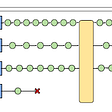## Review — PIRL: Pretext-Invariant Representations Learning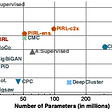## Object Detection Explained: FCOS